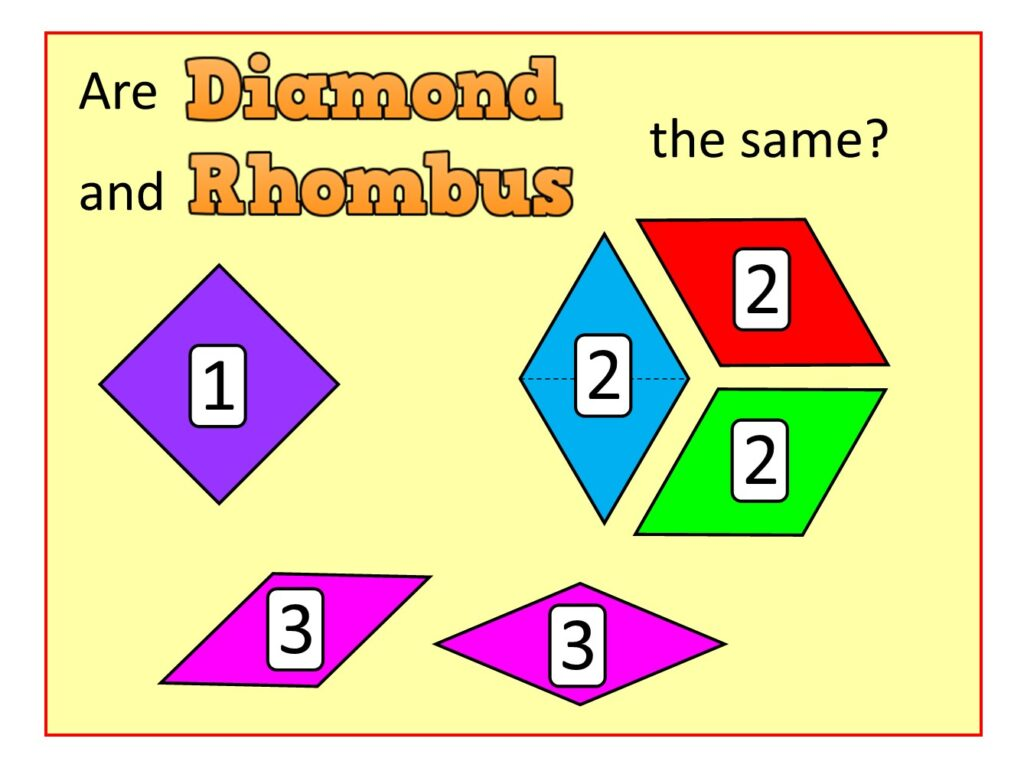07899 756585

# House of Maths School Workshops Primary & Secondary in Dorset & South - Are DIAMOND and RHOMBUS the same?

ALL OF THE SHAPES IN THIS IMAGE ARE RHOMBI (another word for “rhombusses”) BUT ONLY THOSE LABELLED 2 ARE DIAMONDS.In the image: shape number 1 (purple) is a Square (not a diamond), because it is a quadrilateral (4 sides) with four $90^{\circ}$ right-angles. The fact that it is “turned around and balanced on a corner” does not change the fact that it’s a square. Non-mathematicians will often say this is a “diamond” because it’s balanced on a corner. But it’s not a diamond: it’s a square!

DIAMOND: The shapes labelled number 2 (blue, red, green) are all Diamonds, because they are each made up of two equilateral (all sides the same length) triangles stuck together. I’ve emphasised this by drawing a dotted line between the two equilateral triangles in the blue diamond.

Notice how the three diamonds if we squished them together would form a regular hexagon – which is of course made up of six equilateral triangles placed together.

POLYIAMONDS: A Diamond is a special type of Polyiamond (“many triangles”) – the collective name for shapes made up of one or more equilateral triangles stuck together base to base. If you wanted to you could say that a single equilateral triangle is a moniamond (one triangle). The sequence then goes diamond (two triangles), triamond (three), tetriamond (4), pentiamond (5) and so on. There are 3, 4, 12 different types of Tetriamond, Pentiamond, Hexiamond because of the different ways in which you can stick 4, 5, 6 triangles together, and it’s fun to try to sketch them all: have a go!

RHOMBUS (“wonky square”): The shapes labelled number 3 are both rhombi, because they are quadrilaterals whose four sides are all equal in length. The same is true for all the shapes 1, 2 and 3, so they are all rhombi, but only 1 is a square and only 2 are diamonds.

SUMMARY: All Diamonds are Rhombi, but not all Rhombi are Diamonds.

For more on strange shapes, see here for some non-squares that are all pretending to be squares !!